### Multiple Degree of Freedom Systems: Eigenvalue Problem

The problem of finding the natural frequencies and mode shapes for a multiple degree of freedom system is essentially an eigenvalue problem, although we have so far not presented it as such. To see this recall that the equations of motion for an MDOF system can be written as

(8.26)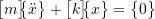where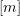and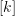are the mass and stiffness matrices respectively and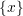is a column vector containing the coordinates. We look for solutions in which all of the coordinates are undergoing simple simultaneous harmonic motion of the form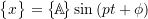so that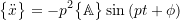Substituting these results into the equations of motion gives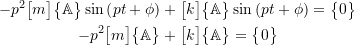or

(8.27)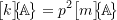Pre-multiplying both sides of (8.27) by the inverse of the mass matrix gives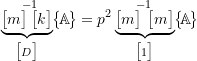or

(8.28)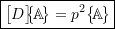which is a standard eigenvalue problem

•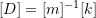is known as the dynamic matrix
• the natural frequencies (squared)are the eigenvalues of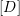• the mode shapes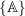are the associated eigenvectors ofThe dynamic matrixcan be used with standard software packages such as Matlab.

Alternatively, starting with equation (8.27) and premultiplying both side by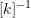gives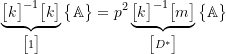or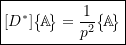which is another formulation of the standard eigenvalue problem.